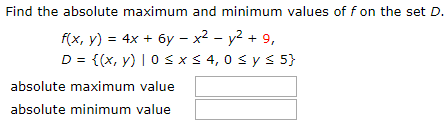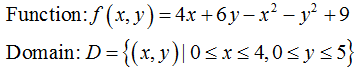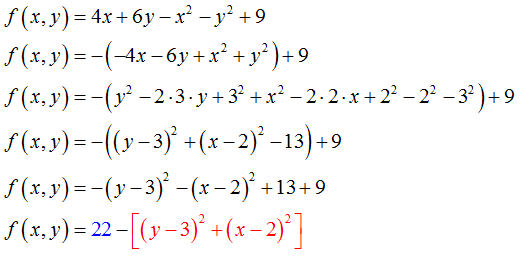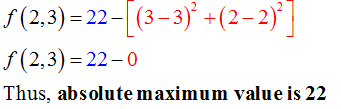# Find the absolute maximum and minimum values of f on the set D.f(x, y) = 4x + 6y – x² – y2 + 9,D = {(x, y) | 0 s xs 4, 0 s ys 5}absolute maximum valueabsolute minimum value%3!

Question
16 viewshelp_outlineImage TranscriptioncloseFind the absolute maximum and minimum values of f on the set D. f(x, y) = 4x + 6y – x² – y2 + 9, D = {(x, y) | 0 s xs 4, 0 s ys 5} absolute maximum value absolute minimum value %3! fullscreen
check_circle

Step 1

Given function and its set of domain is as below.Step 2

Complete the square on the right side of the function as shown below.From the above function it is clear that the term in the square brackets will always greater than or equal to 0.

Step 3

For maximum value:

The function has maximum value if the terms in the braces have least value. The least value of (x – 2)2 and (y – 3)2 is when x = 2 and y = 3....

### Want to see the full answer?

See Solution

#### Want to see this answer and more?

Solutions are written by subject experts who are available 24/7. Questions are typically answered within 1 hour.*

See Solution
*Response times may vary by subject and question.
Tagged in